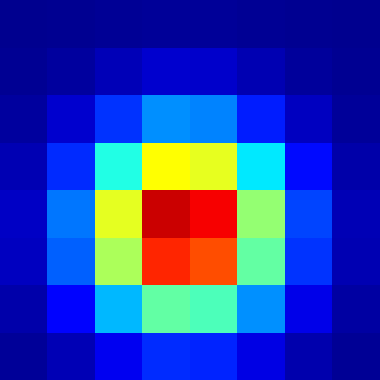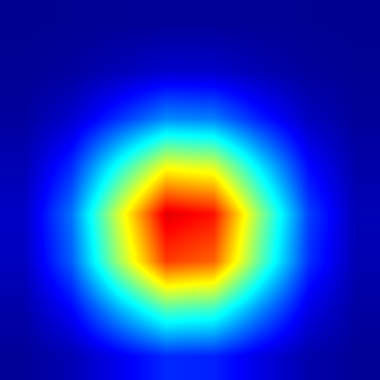# High-order interpolation

Higher-order interpolation can be more appealing altough it is approx. 15 times more expensive than regular interpolate() in 3D.Cell-centered colouringquarticly interpolated colouring

#include "grid/octree.h"
#include "higher-order.h"

#define interpolate interpolate_5
#define quartic linear
#include "utils.h"

scalar s[];

int main() {
L0 = 4;
origin (-L0/2.05, -L0/2.5, -L0/3.);
init_grid (8);
foreach()
s[] = exp(-sq(x) - sq(y) - sq(z));
boundary({s});
output_ppm (s, file = "sn.png", n = 380, max = 1);
output_ppm (s, file = "si.png", n = 380, quartic = true, max = 1);
}